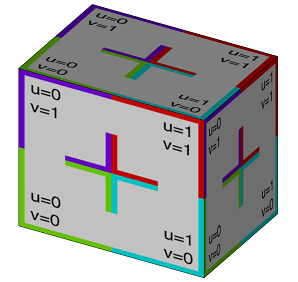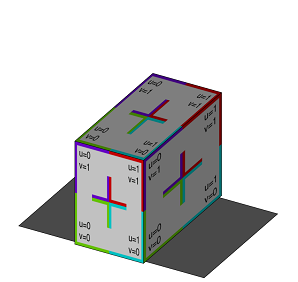# primitiveCube operation

### Syntax

• primitiveCube()
• primitiveCube(width, height, depth)

### Parameters

1. widthfloat
Width of the cube.
2. heightfloat
Height of the cube.
3. depthfloat
Depth of the cube.

### Description

The primitiveCube operation inserts a cube geometry into the scope of the current shape.

• If no width, height and depth are given the bounding box coincides with the scope. Zero scope sizes are handled the same way as in the i operation.
• If dimensions are specified the cube is positioned in the xz-center of the scope lying on y=0.

The cube has texture coordinates on the first texture layer (COLORMAP).

### Examples

 The inserted cube is fit into the current scope. The scope's zero size is modified relative to the average of the two non-zero sizes. ``````Lot--> primitiveCube() texture("builtin:uvtest.png")``````The inserted cube has a width of 10, a height of 15, a depth of 20 and is positioned in the xz center. ``````Lot--> primitiveCube(10,15,20) texture("builtin:uvtest.png")``````